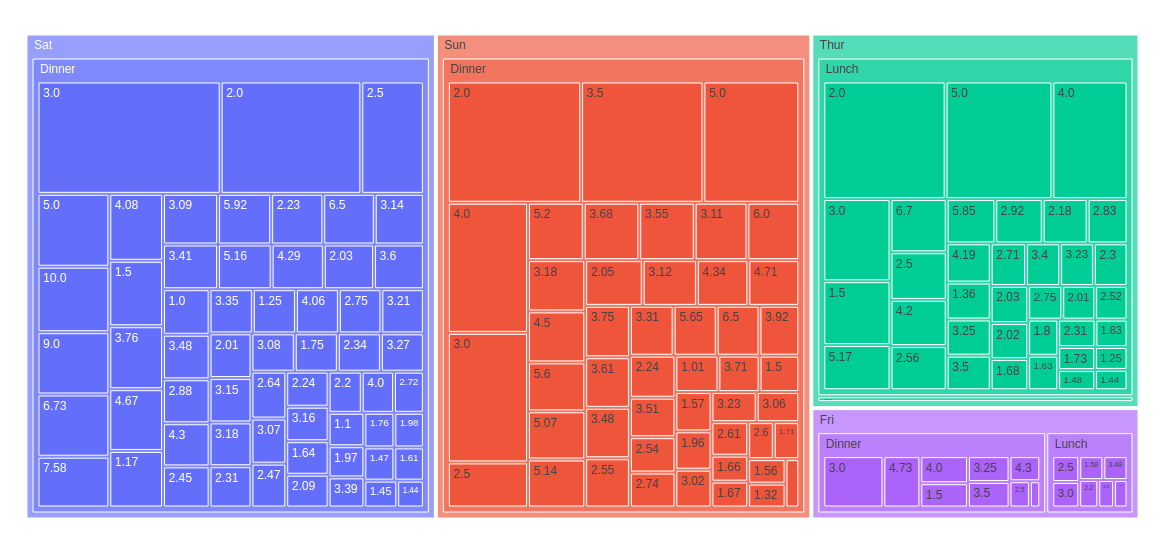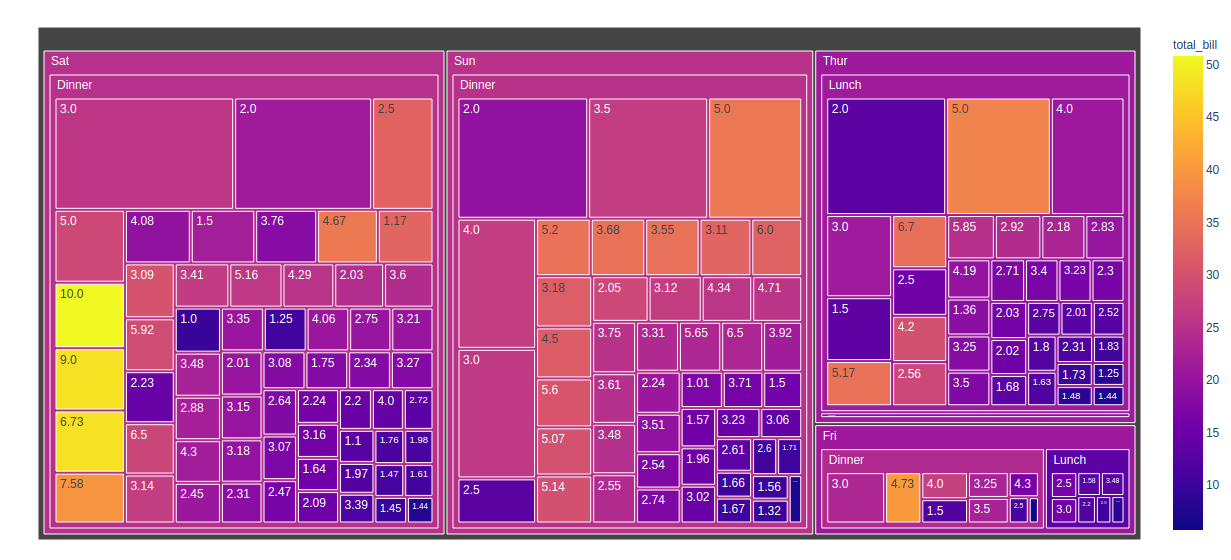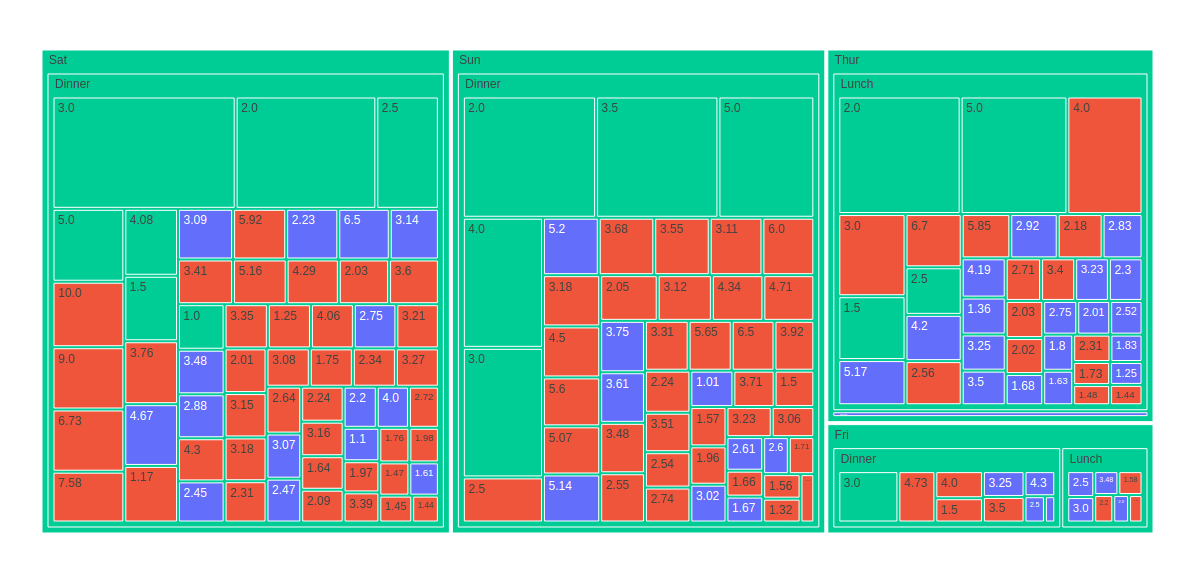GFG App
Open AppBrowser
Continue

# Treemap using Plotly in Python

Plotly is a Python library that is used to design graphs, especially interactive graphs. It can plot various graphs and charts like histogram, barplot, boxplot, spreadplot, and many more. It is mainly used in data analysis as well as financial analysis. plotly is an interactive visualization library.

## Treemap in Plotly

Treemap in plotly.express is convenient to use, high_ranking terminal to plotly, which accomplishes a variety of types of data and produces easy-to-style figures. A treemap provides a stratified view of data and makes it easy to blot the patterns. The tree branches are characterized by rectangles and each of the sub_branches is shown in a smaller rectangle.

Syntax: plotly.express.treemap(data_frame=None, names=None, values=None, parents=None, ids=None, path=None, color=None, color_continuous_scale=None, range_color=None, color_continuous_midpoint=None, color_discrete_sequence=None, color_discrete_map={}, hover_name=None, hover_data=None, custom_data=None, labels={}, title=None, template=None, width=None, height=None, branchvalues=None, maxdepth=None)

Parameters:

data_frame: This argument needs to be passed for column names (and not keyword names) to be used. Array-like and dict are transformed internally to a pandas DataFrame.

names: Either a name of a column in data_frame, or a pandas Series or array_like object. Values from this column or array_like are used as labels for sectors.

values: Either a name of a column in data_frame, or a pandas Series or array_like object. Values from this column or array_like are used to set values associated to sectors.

path: Either names of columns in data_frame, or pandas Series, or array_like objects List of columns names or columns of a rectangular dataframe defining the hierarchy of sectors, from root to leaves.

color: Either a name of a column in data_frame, or a pandas Series or array_like object. Values from this column or array_like are used to assign color to marks.

Example:

## Python3

 `import` `plotly.express as px`     `fig ``=` `px.treemap(` `    ``names ``=` `[``"A"``,``"B"``, ``"C"``, ``"D"``, ``"E"``],` `    ``parents ``=` `["``", "``A``", "``B``", "``C``", "``A"]` `)`   `fig.show()`

Output:## Plotting Treemap of a Hierarchical DataFrame

Rectangular data frames are often stored in Hierarchical form, in which the columns are different corresponding to different levels of the hierarchy. px.treemap can use path parameter which corresponding to a list of columns, but id and parent should be provided if the path is used already.

Example:

## Python3

 `Python3`   `import` `plotly.express as px`   `df ``=` `px.data.tips()`   `fig ``=` `px.treemap(df, path``=``[``'day'``, ``'time'``, ``'tip'``],` `                 ``values``=``'total_bill'``)`   `fig.show()`

Output:#### Plotting Hierarchical dataframe with the continuous color argument

If the color argument are passed, the average of the color values of its children, weighted by their values should be computed by color of node.

Example:

## Python3

 `import` `plotly.express as px`   `df ``=` `px.data.tips()`   `fig ``=` `px.treemap(df, path``=``[``'day'``, ``'time'``, ``'tip'``], ` `                 ``values``=``'total_bill'``, ` `                 ``color``=``'total_bill'``)`   `fig.show()`

Output:#### Plotting Hierarchical dataframe with the discrete color argument

When the non-numeric data corresponds with the color argument, then discrete color are used. If the value of the color column for all the sector is same, then the corresponding color are used, otherwise, the first color of discrete color in the sequence is used.

Example:

## Python3

 `import` `plotly.express as px`   `df ``=` `px.data.tips()`   `fig ``=` `px.treemap(df, path``=``[``'day'``, ``'time'``, ``'tip'``],` `                 ``values``=``'total_bill'``,` `                 ``color``=``'sex'``)`   `fig.show()`

Output:My Personal Notes arrow_drop_up